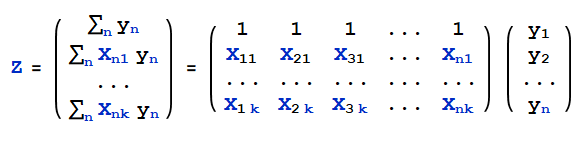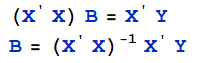# MMSE 学习笔记

*欢迎转载,请注明出处*

Note Author : yanbin chen

Email: yanbin_c@hotmail.com

Reference book

MMSE definition.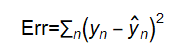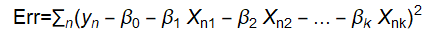With given data, Err is β’s non-negative 2nd order function. It’s minimum value is exist.

The partial derivative should be zero for error minimization.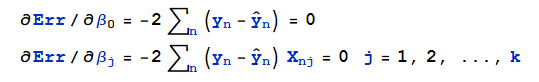This is normal equation.  can be written in following format.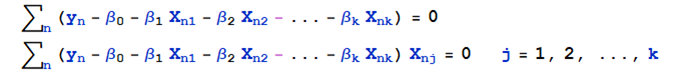Again. expend the equation.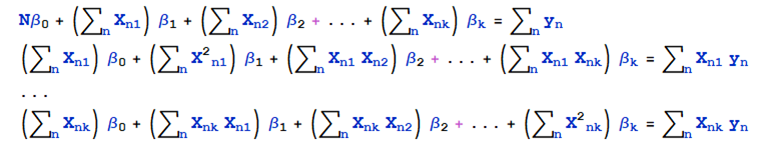Left side coefficient Matrix is symmetric matrices  A=X' X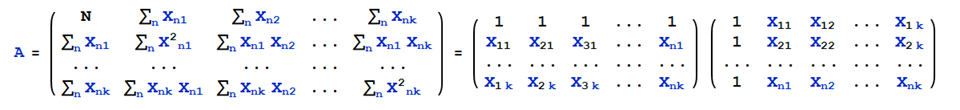Right side constant item Z can be expressed in   Z=X' Y